Resources: Tensegrity
Icosahedron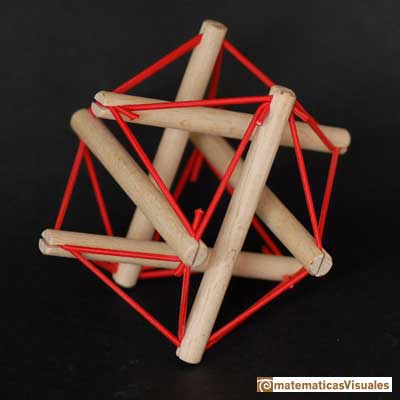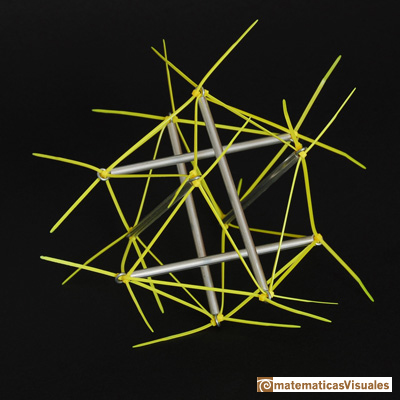The following construction is a mix between the traditional building of an icosahedron with three golden rectangles and a tensegrity. We used cardboard from a tetrabrik because during the pandemic it was not easy to find other matherials.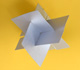A proposal to build an icosahedron using cardboard from a tetrabrik. (Lots of images).

In the following tensegrity I print the vertices of the golden rectangles using a 3d printer. The three rectangles were made using plastic tubes.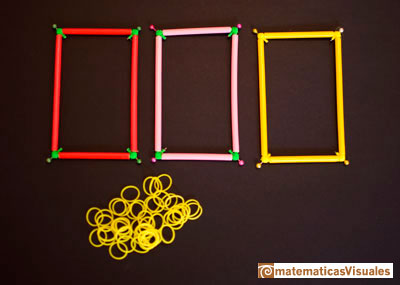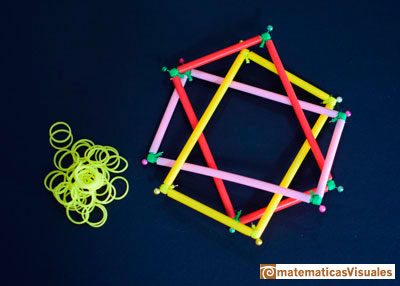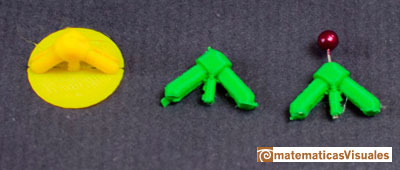More constructions of the icosahedron in the following link:

Cuboctahedron

The cuboctahedron has four hexagonal sections. The twelve vertices of the cuboctahedron are vertices of these four hexagons.A cuboctahedron is an Archimedean solid. It can be seen as made by cutting off the corners of a cube.A cuboctahedron is an Archimedean solid. It can be seen as made by cutting off the corners of an octahedron.

We can consider four equilateral triangles that contains the twelve vertices of the cuboctahedron.

Then, we can build the following tensegrity: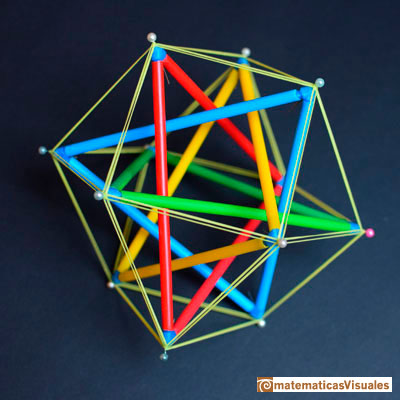I used a 3d printer to build the vertices and plastic tubes for the edges.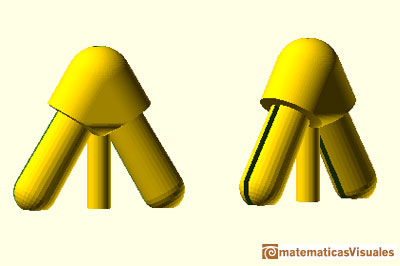Five tetrahedra inscribed in a dodecahedron

We can inscribe five tetrahedra in a dodecahedron and build the following tensegrity: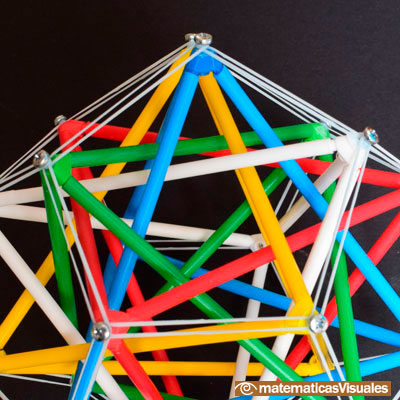This beautiful structure can be built using more techniques. The relation between the dodecahedron, the cube and the tetrahedron can justify why this construction is possible.Different techniques to build five tetraedra inside a dodecahedron: carboard, origami, tensegrity.
Tetrahedron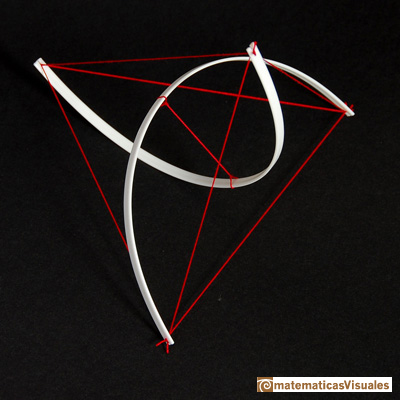I found this model in the wonderful site of Marcelo Pars about tensegrity.

More tensegrities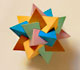Using cardboard you can build beautiful polyhedra cutting polygons and glue them toghether. This is a very simple and effective technique. You can download several templates. Then print, cut and glue: very easy!Simple technique to build polyhedra gluing discs made of cardboard or paper.Italian designer Bruno Munari conceived 'Acona Biconbi' as a work of sculpture. It is also a beautiful game to play with colors and shapes.Material for a session about polyhedra (Zaragoza, 9th May 2014). Simple techniques to build polyhedra like the tetrahedron, octahedron, the cuboctahedron and the rhombic dodecahedron. We can build a box that is a rhombic dodecahedron.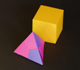Material for a session about polyhedra (Zaragoza, 7th November 2014). We study the octahedron and the tetrahedron and their volumes. The truncated octahedron helps us to this task. We build a cubic box with cardboard and an origami tetrahedron.Material for a session about polyhedra (Zaragoza, 23rd Octuber 2015) . Building a cube with cardboard and an origami octahedron.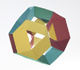Material for a session about polyhedra (Zaragoza, 21st October 2016). Instructions to build several geometric bodies.Microarquitectura is a construction game developed by Sara San Gregorio. You can play and build a lot of structures modelled on polyhedra.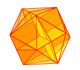The twelve vertices of an icosahedron lie in three golden rectangles. Then we can calculate the volume of an icosahedronThe volume of a tetrahedron is one third of the prism that contains it.The first drawing of a plane net of a regular tetrahedron was published by Dürer in his book 'Underweysung der Messung' ('Four Books of Measurement'), published in 1525 .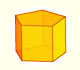We study different prisms and we can see how they develop into a plane net. Then we explain how to calculate the lateral surface area.We study different cylinders and we can see how they develop into a plane. Then we explain how to calculate the lateral surface area.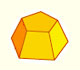Plane net of pyramids and pyramidal frustrum. How to calculate the lateral surface area.Plane developments of cones and conical frustum. How to calculate the lateral surface area.The first drawing of a plane net of a regular dodecahedron was published by Dürer in his book 'Underweysung der Messung' ('Four Books of Measurement'), published in 1525 .